# NCERT Solutions for Class 7 Maths Chapter 3 Data Handling

In this page we have NCERT Solutions for Class 7th Maths Chapter 3: Data Handling . Hope you like them and do not forget to like , social share and comment at the end of the page.

## Exercise 3.1

Question 1
Find the range of heights of any ten students of your class.
Solution
Let the heights (in cm) of 10 students of our class be
125, 127, 132, 133, 134, 136,138, 141, 144, 146
Highest value among these observations = 146
Lowest value among these observations = 125
Range is defined as the difference of highest and lowest value in the data
Range = Highest value − Lowest value
= (146 − 125) cm
= 21 cm
Question 2
Organize the following marks in a class assessment, in a tabular form.
4, 6, 7, 5, 3, 5, 4, 5, 2, 6, 2, 5, 1, 9, 6, 5, 8, 4, 6, 7
(i) Which number is the highest?
(ii) Which number is the lowest?
(iii) What is the range of the data?
(iv) Find the arithmetic mean.
Solution
Let's first convert into tabular form
 Marks Tally marks Frequency 1 | 1 2 || 2 3 | 1 4 ||| 3 7 || 2 8 | 1 9 | 1
Now from the above table, we can easily answer the above questions (i) Highest number = 9
(ii) Lowest number = 1
(iii) Range = (9 − 1) = 8
(iv) Sum of all the observations = 4 + 6 + 7 + 5 + 3 + 5 + 4 + 5 + 2 + 6 + 2
+ 5 + 1 + 9 + 6 + 5 + 8 + 4 + 6 + 7
= 100
Total number of observations = 20
Arithmetic Mean is defined as the ratio of sum of all the observation and total number of observations
$\text{Arithmetic mean}= \frac {(Sum \;of\; all\; the \;obersvation)}{(Total\; Number \;of\; Observations)}= \frac {100}{20}=50$
Question 3
Find the mean of the first five whole numbers.
Solution
We know that First five whole numbers are 0, 1, 2, 3, and 4.
$Mean= \frac {(0+1+2+3+4) } {5}= \frac {10} {5}=2$
Therefore, the mean of first five whole numbers is 2.

Question 4:
A cricketer scores the following runs in eight innings:
58, 76, 40, 35, 46, 45, 0, 100
Find the mean score.
Solution
Runs scored by the cricketer are 58, 76, 40, 35, 46, 45, 0, and 100.
$Mean= \frac {(58+76+40+35+46+45+0+100)} {8}= \frac {400}{8}=50$
Therefore, mean score is 50.
Question 5:
Following table shows the points of each player scored in four games:
 Player Game 1 Game 2 Game 3 Game 4 A 14 16 10 10 B 0 8 6 4 C 8 11 Did not play 13
(i) Find the mean to determine A’s average number of points scored per game.
(ii) To find the mean number of points per game for C, would you divide the total points by 3 or by 4? Why?
(iii) B played in all the four games. How would you find the mean?
(iv) Who is the best performer?
Solution
i) A’s average number of points is given by
$= \frac {(14+16+10+10)}{4}= \frac {50}{4}=12.5$

(ii) To find the mean number of points per game for C, we will divide the total points by 3 because C played 3 games.
iii)
$\text{Mean of B's Score}= \frac {(0+8+6+4)}{4}= \frac {18}{4}=4.5$
iv)  The best performer will have the greatest average among all. Now we can observe that the average of A is 12.5 which is more than that of B and C.
Therefore ,A is the best performer among these three
Question 6:
The marks (out of 100) obtained by a group of students in a science test are 85, 76, 90, 85, 39, 48, 56, 95, 81 and 75. Find the:
(i) Highest and the lowest marks obtained by the students.
(ii) Range of the marks obtained.
(iii) Mean marks obtained by the group.
Solution
The marks obtained by the group of students in a science test can be arranged in an ascending order as follows.
39, 48, 56, 75, 76, 81, 85, 85, 90, 95
(i) Highest marks = 95
Lowest marks = 39
(ii) Range = 95 − 39
= 56
ii)
$\text{Mean Marks}= \frac {(85+76+90+85+39+48+56+95+81+75)}{10}= \frac {730}{10}=73$
Question 7:
The enrolment in a school during six consecutive years was as follow: 1555, 1670, 1750, 2013, 2540, 2820
Find the mean enrolment of the school for this period.
Solution
$\text{Mean Enrolment}= \frac {(1555+1670+1750+2013+2540+2820)}{6}= \frac {12348}{6}=2058$
Question 8:
The rainfall (in mm) in a city on 7 days of a certain week was recorded as follows:
 Days Rain fall (in mm) Monday 0.0 Tuesday 12.2 Wednesday 2.1 Thursday 0.0 Friday 20.5 Saturday 5.5 Sunday 1.0
(i) Find the range of the rainfall in the above data.
(ii) Find the mean rainfall for the week.
(iii) On how many days was the rainfall less than the mean rainfall.
Solution
(i) Range = (20.5 − 0.0) mm
= 20.5 mm
ii)
$\text{Mean Rainfall}= \frac {(0.0+12.2+2.1+0.0+20.5+5.5+1.0)}{7}=\frac {41.3}{7}=5.9mm$
iii) For 5 days (Monday, Wednesday, Thursday, Saturday, Sunday),the rainfall was
less than the average rainfall.
Question 9:
The heights of 10 girls were measured in cm and the results are as follows:
135, 150, 139, 128, 151, 132, 146, 149, 143, 141
(i) What is the height of the tallest girl?
(ii) What is the height of the shortest girl?
(iii) What is the range of the data?
(iv) What is the mean height of the girls?
(v) How many girls have heights more than the mean height.
Solution
Arranging the heights of 10 girls in an ascending order, we can get most of the data
128 >  132 >  135b > 139 >  141 > 143 > 146 > 149 > 150 > 151
(i) Height of the tallest girl = 151 cm
(ii) Height of the shortest girl = 128 cm
(iii) Range = (151 − 128) cm
= 23 cm
iv)
$\text{Mean height}= \frac {(135+150+139+128+151+132+146+149+143+141)}{10}= \frac {1414}{10}=141.4 cm$
v) The heights of 5 girls are greater than the mean height (i.e.,141.4 cm) and these heights are 143,146,149,150,and 151 cm.

## Exercise 3.2

Question 1:
The scores in mathematics test (out of 25) of 15 students is as follows:
19, 25, 23, 20, 9, 20, 15, 10, 5, 16, 25, 20, 24, 12, 20
Find the mode and median of this data. Are they same?
Solution
Scores of 15 students in mathematics test are
19, 25, 23, 20, 9, 20, 15, 10, 5, 16, 25, 20, 24, 12, 20
Arranging these scores in an ascending order,
5, 9, 10, 12, 15, 16, 19, 20, 20, 20, 20, 23, 24, 25, 25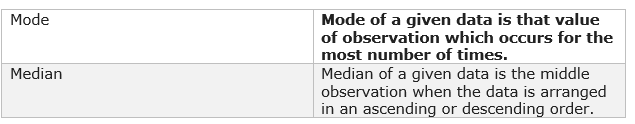As there are 15 terms in the given data, therefore, the median of this data will be the 8th observation.
Hence, median = 20
Also, it can be noticed that 20 occurs 4 times (i.e., maximum number of times).
Therefore, mode of this data = 20
Yes, both are same.
Question 2:
The run scored in a cricket match by 11 players is as follows:
6, 15, 120, 50, 100, 80, 10, 15, 8, 10, 15
Find the mean, mode and median of this data. Are the three same?
Solution
The runs scored by 11 players are
6, 15, 120, 50, 100, 80, 10, 15, 8, 10, 15
Arranging these scores in an ascending order,
6, 8, 10, 10, 15, 15, 15, 50, 80, 100, 120
$Mean=\frac {(6+8+10+10+15+15+15+50+80+100+120)}{11}= \frac {429}{11}=39$As there are 11 terms in the given data, therefore, the median of this data will be the 6th observation.
Median = 15
Also, it can be noticed that 15 occurs 3 times (i.e., maximum number of times).
Therefore, mode of this data = 15
No, these three are not same.
Question 3:
The weights (in kg.) of 15 students of a class are:
38, 42, 35, 37, 45, 50, 32, 43, 43, 40, 36, 38, 43, 38, 47
(i) Find the mode and median of this data.
(ii) Is there more than one mode?
Solution
The weights of 15 students are
38, 42, 35, 37, 45, 50, 32, 43, 43, 40, 36, 38, 43, 38, 47
Arranging these weights in ascending order,
32, 35, 36, 37, 38, 38, 38, 40, 42, 43, 43, 43, 45, 47, 50
(i)As there are 15 terms in the given data, therefore, the median of this data will be the 8th observation.
Hence, median = 40
Also, it can be noticed that 38 and 43 both occur 3 times (i.e., maximum number of times).
hence, mode of this data = 38 and 43
(ii)
Yes, there are 2 modes for the given data.
Question 4:
Find the mode and median of the data: 13, 16, 12, 14, 19, 12, 14, 13, 14
Solution
The given data is
13, 16, 12, 14, 19, 12, 14, 13, 14
Arranging the given data in an ascending order,
12, 12, 13, 13, 14, 14, 14, 16, 19As there are 9 terms in the given data, therefore, the median of this data will be the 5th observation.
Hence, median = 14
Also, it can be noticed that 14 occurs 3 times (i.e., maximum number of times).
hence, mode of this data = 14
Question 5:
Tell whether the statement is true or false:
(i) The mode is always one of the numbers in a data.
(ii) The mean is one of the numbers in a data.
(iii) The median is always one of the numbers in a data.
(iv) The data 6, 4, 3, 8, 9, 12, 13, 9 has mean 9.
Solution
(i) True
Mode of a given data is that value of observation which occurs for the most number of times. Therefore, it is one of the observations given in the data.
(ii) False
Mean may or may not be one of the numbers in the data.
(iii) True
The median of the given data is the middle observation when the data is arranged in an ascending or descending order.
(iv) False
The given data is 6, 4, 3, 8, 9, 12, 13, 9
$Mean=\frac {(6+4+3+8+9+12+13+9)}{8}= \frac {64}{8}=8$

## Exercise 3.3

Question 1:
Use the bar graph (see the given figure) to answer the following questions.
(a) Which is the most popular pet?
(b) How many children have dog as a pet?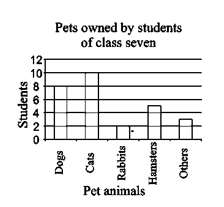Solution
(a) Since the bar representing cats is the tallest, cat is the most popular pet.
(b) The number of children having dog as a pet are 8.
Question 2:
Read the bar graph (see the given figure) which shows the number of books sold by a bookstore during five consecutive years and answer the questions that follow: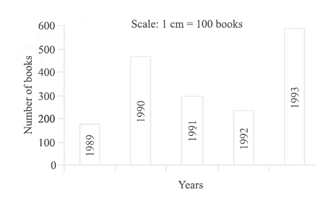(i) About how many books were sold in 1989? 1990? 1992?
(ii) In which year were about 475 books sold? About 225 books sold?
(iii) In which years were fewer than 250 books sold?
(iv) Can you explain how you would estimate the number of books sold in 1989?
Solution
(i) In 1989, 175 books were sold. In 1990, 475 books were sold. In 1992, 225 books were sold.
(ii) From the graph, it can be concluded that 475 books were sold in the year 1990 and 225 books were sold in the year 1992.
(iii) From the graph, it can be concluded that in the years 1989 and 1992, the number of books sold were less than 250.
(iv) From the graph, it can be concluded that the number of books sold in the year 1989 is about 1 and ¾ th  part of 1 cm.
We know that the scale is taken as 1 cm = 100 books.
100 + (3/4) X100=175

Therefore, about 175 books were sold in the year 1989.
Question 3:
Number of children in six different classes are given below. Represent the data on a bar graph.
 Class Fifth Sixth Seventh Eighth Ninth Tenth Number of children 135 120 95 100 90 80
(a) How would you choose a scale?
(i) Which class has the maximum number of children? And the minimum?
(ii) Find the ratio of students of class sixth to the students of class eight.
Solution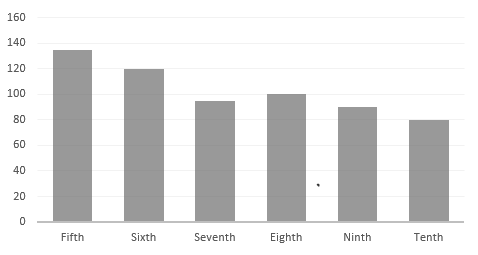(a) We will choose a scale as 1 unit = 10 children because we can represent a more clear difference between the number of students of class 7th and that of class 9th by this scale.
(b)
(i) Since the bar representing the number of children for class fifth is the tallest, there are maximum number of children in class fifth. Similarly, since the bar representing the number of children for class tenth is the smallest, there are minimum number of children in class tenth.
(ii) The number of students in class sixth is 120 and the number of students in class eighth is 100.
Therefore, the ratio between the number of students of class sixth and the number of
Student of Class 8th =120/100 =6:5

Question 4:
The performance of students in 1st Term and 2nd Term is given. Draw a double bar graph choosing appropriate scale and answer the following:
 Subject English Hindi Maths Science S. science 1st Term (M.M. 100) 67 72 88 81 73 2nd Term (M.M. 100) 70 65 95 85 75
(i) In which subject, has the child improved his performance the most?
(ii) In which subject is the improvement the least?
(iii) Has the performance gone down in any subject?
Solution
A double bar graph for the given data is as follows.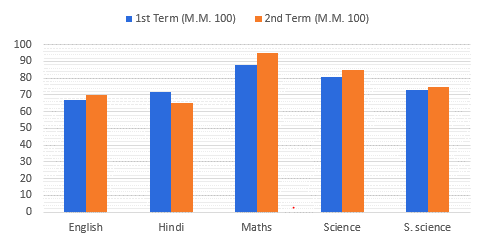(i) There was a maximum increase in the marks obtained in Maths. Therefore, the child has improved his performance the most in Maths.
(ii) From the graph, it can be concluded that the improvement was the least in S. Science.
(iii) From the graph, it can be noticed that the performance in Hindi has gone down.
Question 5:
Consider this data collected from a survey of a colony.
 Favorite sport Cricket Basket Ball Swimming Hockey Athletics Watching 1240 470 510 430 250 Participating 620 320 320 250 105
(i) Draw a double bar graph choosing an appropriate scale. What do you infer from the bar graph?
(ii) Which sport is most popular?
(iii) Which is more preferred, watching or participating in sports?
Solution
(i)A double bar graph for the given data is as follows.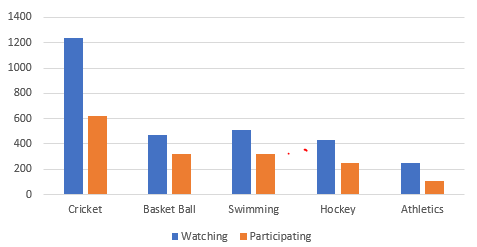The double bar graph represents the number of people who like watching and participating in different sports. It can be noticed that most of the people like watching and participating in cricket while the least number of people like watching and participating in athletics.
(ii) From the bar graph, it can be noticed that the bar representing the number of people who like watching and participating in cricket is the tallest among all the bars. Hence, cricket is the most popular sport.
(iii) The bars representing watching sport are longer than the bars representing participating in sport. Hence, watching different types of sports is more preferred than participating in the sports.
Question 6:
Take the data giving the minimum and the maximum temperature of various cities given in the following table:
Temperatures of the cities as on 20.6.2006
 City Max. Min. Ahmedabad 38 ºC 29 ºC Amritsar 37 ºC 26 ºC Bangalore 28 ºC 21 ºC Chennai 36 ºC 27 ºC Delhi 38 ºC 28 ºC Jaipur 39 ºC 29 ºC Jammu 41 ºC 26 ºC Mumbai 32 ºC 27 ºC
Plot a double bar graph using the data and answer the following:
(i) Which city has the largest difference in the minimum and maximum temperature on the given date?
(ii) Which is the hottest city and which is the coldest city?
(iii) Name two cities where maximum temperature of one was less than the minimum temperature of the other.
(iv) Name the city which has the least difference between its minimum and the maximum temperature.
Solution
A double bar graph for the given data is constructed as follows.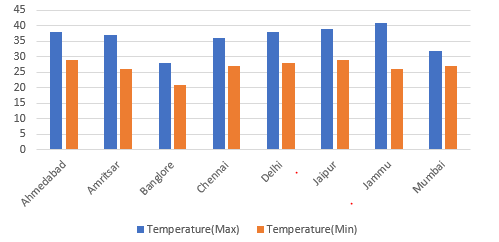(i) From the graph, it can be concluded that Jammu has the largest difference in its minimum and maximum temperatures on 20.6.2006.
(ii) From the graph, it can be concluded that Jammu is the hottest city and Bangalore is the coldest city.
(iii) Bangalore and Jaipur, Bangalore and Ahmedabad
For Bangalore, the maximum temperature was 28ºC, while minimum temperature of both cities, Ahmedabad and Jaipur, was 29ºC.
(iv) From the graph, it can be concluded that the city which has the least difference between its minimum and maximum temperatures is Mumbai.

## Chapter 3 Exercise 3.4

Question 1
Tell whether the following is certain to happen, impossible, can happen but not certain.
(i) You are older today than yesterday.
(ii) A tossed coin will land heads up.
(iii) A die when tossed shall land up with 8 on top.
(iv) The next traffic light seen will be green.
(v) Tomorrow will be a cloudy day.
Solution
(i) Certain
(ii) Can happen but not certain
iii. Impossible as there are only six faces on a dice marked as 1, 2, 3, 4, 5, 6 on it.
(iv) Can happen but not certain
(v) Can happen but not certain
Question 2
There are 6 marbles in a box with numbers from 1 to 6 marked on each of them.
(i) What is the probability of drawing a marble with number 2?
(ii) What is the probability of drawing a marble with number 5?
Solution
i)
$Probability= \frac {\text{Number of Favourable outcomes}}{\text{Number of Possible outcomes}}$
P (Drawing a marble of 2) =1/6
ii) P (Drawing a marble of 5) =1/6
Question 3
A coin is flipped to decide which team starts the game. What is the probability that your team will start?
Solution
A coin has two faces − Head and Tail. One team can opt either Head or Tail.
$Probability= \frac {\text{Number of Favourable outcomes}}{\text{Number of Possible outcomes}}$
Probability of our team starts =1/2

Question 4
A box contains pairs of socks of two colors (black and white). I have picked out a white sock. I pick out one more with my eyes closed. What is the probability that it will make a pair?
Solution
It can be noticed that while closing the eyes, one can draw either a black sock or a white sock. Therefore, there are two possible cases.
$Probability= \frac {\text{Number of Favourable outcomes}}{\text{Number of Possible outcomes}}$
Probability (a pair of white socks will be formed) =1/2

## Similar type of Questions for Practice

1) Find the mean
a) 3,4,5,6,7,8,9,10
b) 1.5,1.2,2.3,2.4,3.5
c) the mean of your sleeping hours during one week.

2) Find the mode of the given set of numbers
a) 1, 1, 1, 2, 2, 2, 2, 3, 4, 4,5,4,5,6,6,7
b) 35,26,35,66,35,56,26,26,34,24
c) 2, 2, 2, 3, 3, 4, 5, 5, 5, 6, 6, 8

3) Find the median of the data
a) 15,10,12,18,14,16,7
b) 1,7,4,2,3,4
c) 16, 24, 20, 39, 19, 23, 26, 23, 18, 20